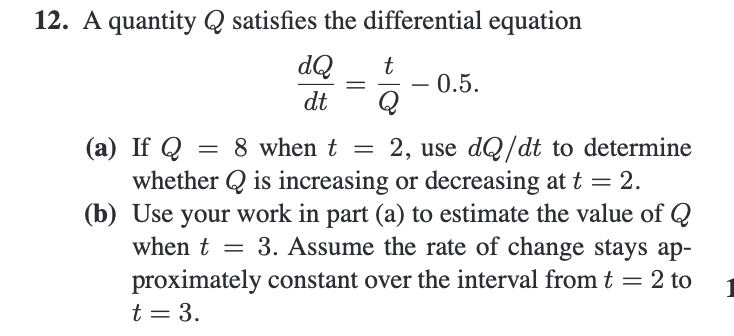Home / Expert Answers / Calculus / 12-a-quantity-q-satisfies-the-differential-equation-dtdq-qt0-5-a-if-q-8-when-t-2-use-pa169

# (Solved): 12. A quantity Q satisfies the differential equation dtdQ=Qt0.5. (a) If Q=8 when t=2, use ...12. A quantity satisfies the differential equation (a) If when , use to determine whether is increasing or decreasing at . (b) Use your work in part (a) to estimate the value of when . Assume the rate of change stays approximately constant over the interval from to .

We have an Answer from Expert